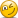Question on Value at Risk (VaR). 2007 FRM, Part 7: Cash Flow at Risk (

Liming

New Member
Dear David,

I have two questions after reading the above-mentioned page (http://www.bionicturtle.com/learn/article/value_at_risk_var_2007_frm_part_7_cash_flow_at_risk_cfar/)

please kindly correct me if I am wrong:

1) When you say that :"For example, assume our company's expected cash flow is $10 million with a volatility of$1 million. Further, assume we consider cash flow below $8 million to be a shortfall. Then we can say either:Our 95% CFaR is$8.36 million: $10 million - ($1 million x 1.645) = $8.36 million", I think here CFVAR refers to absolute VAR.For example, if sigma is 8 million instead of 1 million, then 95% CFaR is 3.16 million: (8 x 1.645) - 10 = 3.16 million. 2) when you earlier give the formula of CAR: CFAR= Prob(E(c) - C > CAR) = p% And here we are measuring Cash flow CAR as the relative VAR, with the p% as the significance level, C as realized cash flow, E(c) as expected cash flow. So in my input, that means CFAR = 10 - (-3.16) = 13.16 million, Thank you very much for your correction! Cheers! Liming David Harper CFA FRM David Harper CFA FRM Staff member Subscriber Hi Liming, Yes, excellent excellent observation! Unfortunately, GARP has not standardized on a definition of CFaR (aka, Stulz CaR which has been replaced by Dowd's LaR), but I agree with your characterization; i.e., if E(C) 0=$10 and cash flow volatlity = $8, then "relative" CFaR = 8*1.645 = 13.16, and for "absolute" it would be okay to follow Stulz with: "absolute" CFaR = 10 - 13.16 = -3.16 ... but yours is even more precise: -E(C) + (8*1.645) = loss (cash outflow) of 3.16 i.e., a positive absolute CFaR represents cash outflow (rather than an inflow) "relative" to zero very helpful, I hope others will look at your example! David [email protected] New Member Dear David, I am currently writing my master thesis on currency hedging. The CFaR measure is therefore of interest to me. I dropped by this page, and read the article that Liming referred to above. However, the formulas seem to be missing. Is it possible for you to put them back into the article somehow? Kind regards, Joakim David Harper CFA FRM David Harper CFA FRM Staff member Subscriber Hi Joakim, I'm not planning on restoring old images like that (we are in process for a major upgrade). sorry.... But the formula was from the (currently unassigned in 2010 FRM) Stulz Chapter 4: Prob [ E(C) - C > CFaR] = significance% (Stulz Risk Management & Derivatives, page 90). Here is link but i do not recommend this book, it is one of my least favorite FRM assignments due to its confusing presentations. ... and this corresponds to a so-called "relative" VaR/CFaR meaning the measure at risk is relative to the future expected E(C) and so Stulz' definition would produce, if say cash flow volatility is$10 MM and normal, a CFaR of $10*1.645; i.e., 5% of the time, we'd expect normally distributed flows to be worse than$10*1.645 from their expected (average) value.
... so, I didn't like Stulz approach b/c he's not totally clear on the three different ways we can define shortfall: relative to expected cash flow E(C), relative to zero/time 0 (i.e., a loss), or relative to funding threshold. It's the same definitional issue (IMO) as pension surplus at risk (SaR). My point is that the formula above, you can see the CFaR is defined as a "a cash flow shortfall relative to the average expected shortfall, E(C)" but it does not need to be defined that way.

Thanks, David

Hope that helps!

southeuro

Member
Hi David,

Hope all's wel.

Something picked my interest: In comparing Var with Lar - shouldn't "short-term exposure on a portfolio of long Euro-style options" be the same as "long-term exposure on a portfolio of short Euro-style options"? Aren't in both cases we have Var exceeding Lar? options pay-offs are not until the end of maturity and no margin calls as you'd have in futures contracts, so no cash flow (low Lar). And if I were to compare the 2 for Var, I'd tend to think that the 2nd position, a long-term exposure and being short actually would result in more Var -- reasoning being, the longer the time of exposure the higher the volatility (hence the Var).

Thanks & cheers,

hamu4ok

Active Member
Hi Liming,

Yes, excellent excellent observation! Unfortunately, GARP has not standardized on a definition of CFaR (aka, Stulz CaR which has been replaced by Dowd's LaR), but I agree with your characterization; i.e., if E(C) 0= $10 and cash flow volatlity =$8,

then "relative" CFaR = 8*1.645 = 13.16, and

for "absolute" it would be okay to follow Stulz with:
"absolute" CFaR = 10 - 13.16 = -3.16

... but yours is even more precise:
-E(C) + (8*1.645) = loss (cash outflow) of 3.16
i.e., a positive absolute CFaR represents cash outflow (rather than an inflow) "relative" to zero

Personally, I believe Stulz reading is still on FRM, only due to the fact that the author is (was) on board of directors of GARP,like Jorion. A bit critisism on them, as they try to use their powers to boost sales of their own books. But it seems GARP is changing now, different authors and very interesting readings are assigned (Gregory, Tuckman, Dowd, etc)